# Chemical Reactions Types of Chemical Reactions I II

• Slides: 22Chemical Reactions Types of Chemical Reactions I II IV V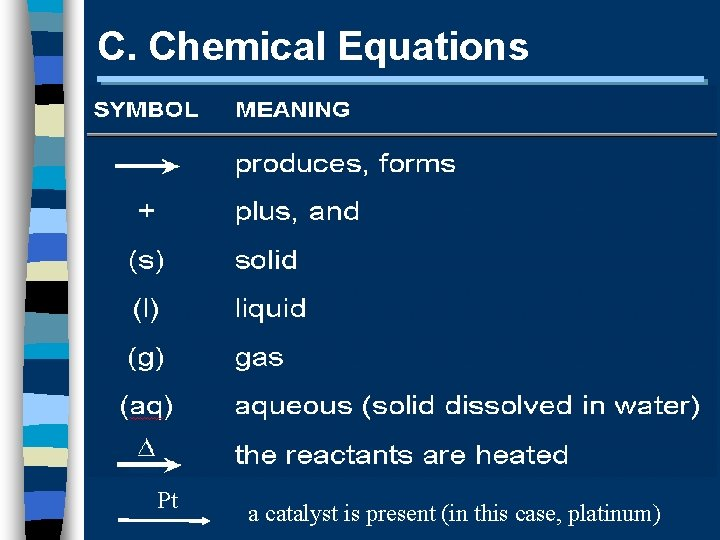C. Chemical Equations Pt a catalyst is present (in this case, platinum)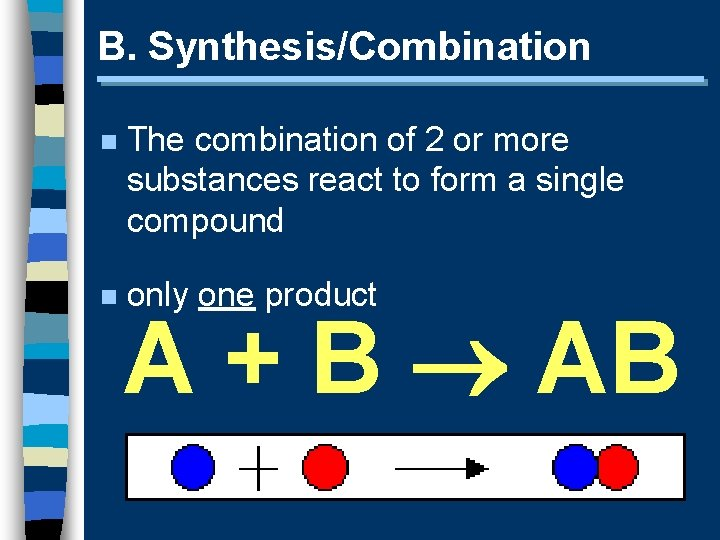B. Synthesis/Combination n The combination of 2 or more substances react to form a single compound n only one product A + B AB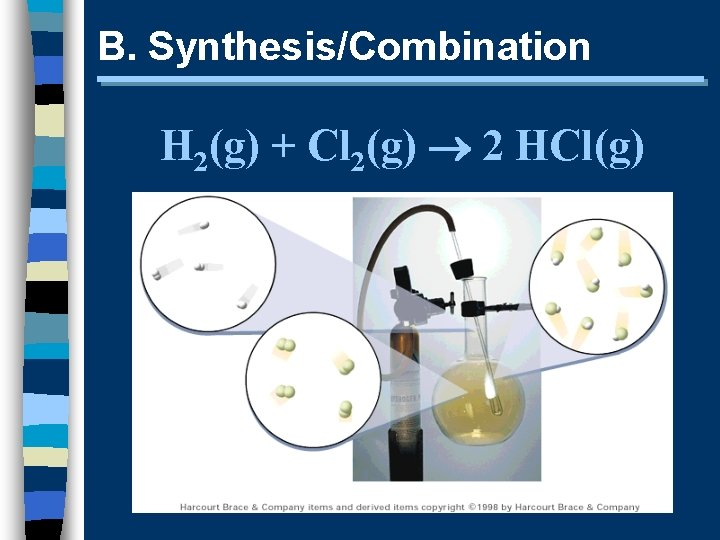B. Synthesis/Combination H 2(g) + Cl 2(g) 2 HCl(g)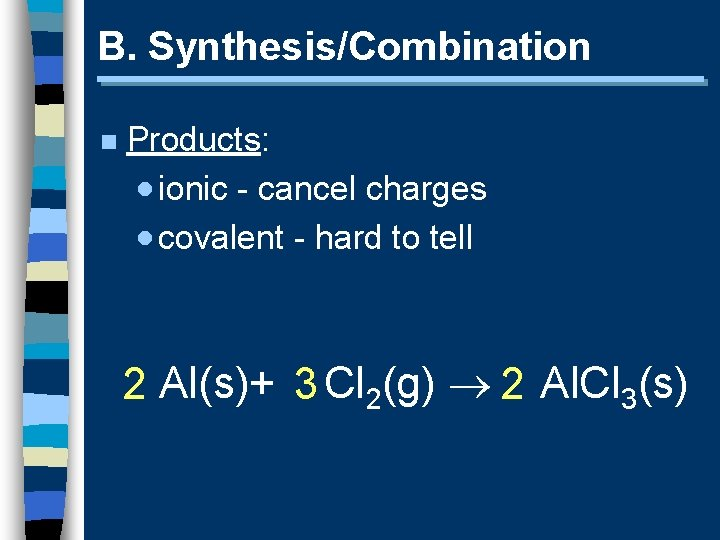B. Synthesis/Combination n Products: · ionic - cancel charges · covalent - hard to tell 2 Al(s)+ 3 Cl 2(g) 2 Al. Cl 3(s)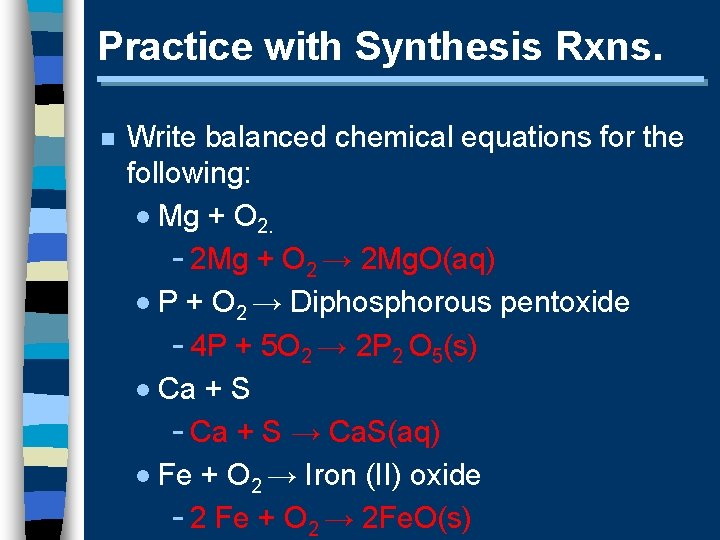Practice with Synthesis Rxns. n Write balanced chemical equations for the following: · Mg + O 2. - 2 Mg + O 2 → 2 Mg. O(aq) · P + O 2 → Diphosphorous pentoxide - 4 P + 5 O 2 → 2 P 2 O 5(s) · Ca + S - Ca + S → Ca. S(aq) · Fe + O 2 → Iron (II) oxide - 2 Fe + O 2 → 2 Fe. O(s)C. Decomposition n a compound breaks down into 2 or more simpler substances n only one reactant AB A + BC. Decomposition 2 H 2 O(l) 2 H 2(g) + O 2(g)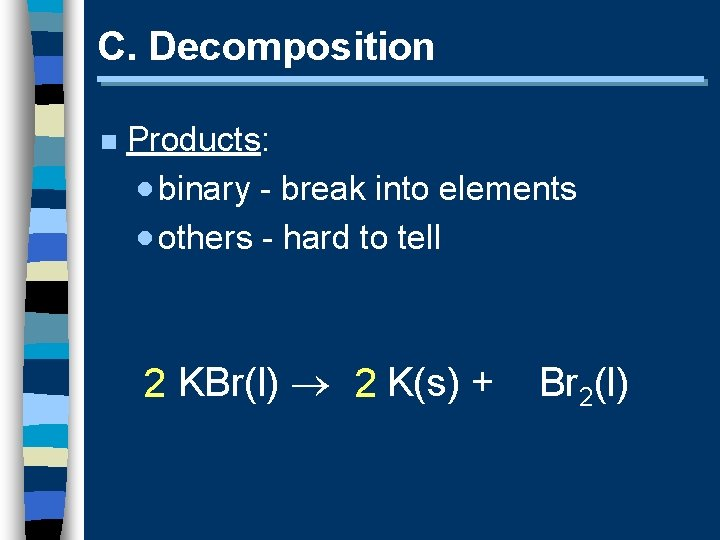C. Decomposition n Products: · binary - break into elements · others - hard to tell 2 KBr(l) 2 K(s) + Br 2(l)Practice with Decomposition Rxns. n Write balanced chemical equations for the following: · HBr - 2 HBr → H 2(g) + Br 2(g) · Na. Cl - 2 Na. Cl → 2 Na(s) + Cl 2(g) · Ag 2 O - 2 Ag 2 O → 4 Ag(s) + O 2(g) · Mercury (II) oxide - 2 Hg. O → 2 Hg(l) + O 2(g)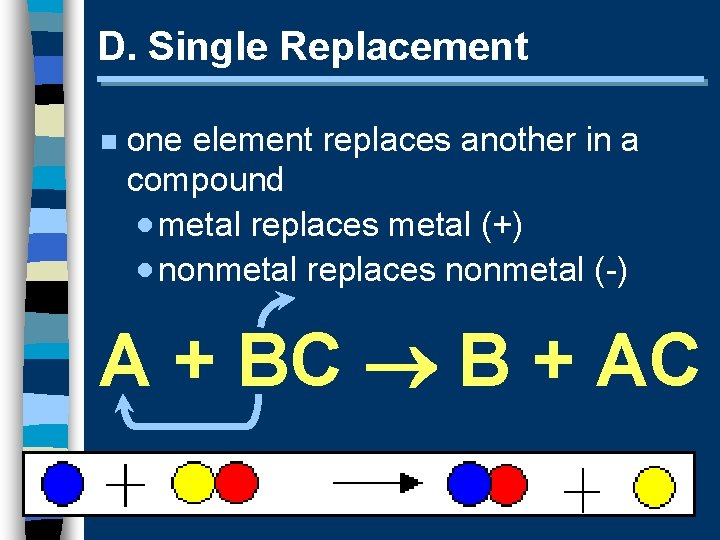D. Single Replacement n one element replaces another in a compound · metal replaces metal (+) · nonmetal replaces nonmetal (-) A + BC B + AC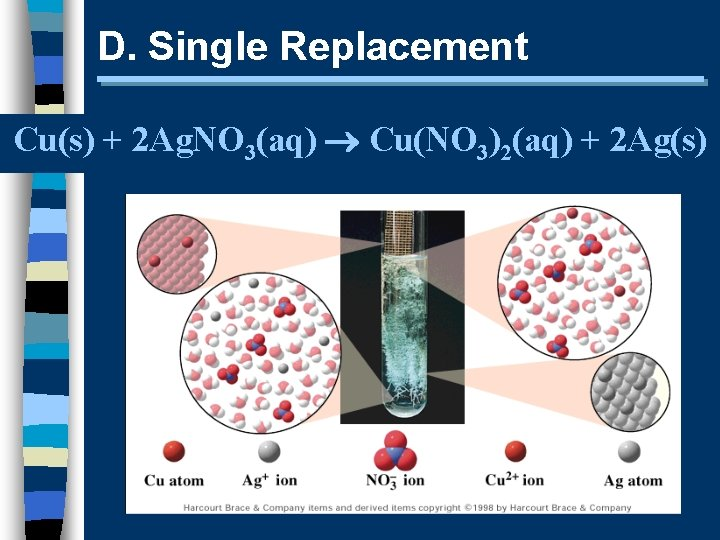D. Single Replacement Cu(s) + 2 Ag. NO 3(aq) Cu(NO 3)2(aq) + 2 Ag(s)D. Single Replacement n Products: · metal (+) · nonmetal (-) · free element must be more active (check activity series)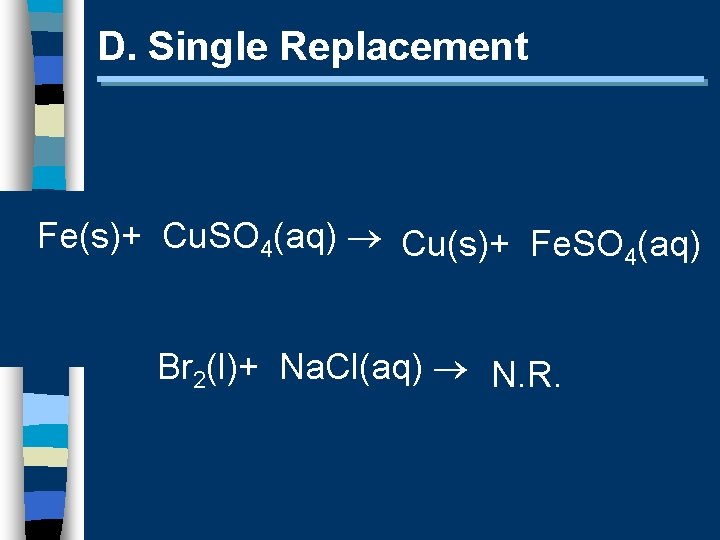D. Single Replacement Fe(s)+ Cu. SO 4(aq) Cu(s)+ Fe. SO 4(aq) Br 2(l)+ Na. Cl(aq) N. R.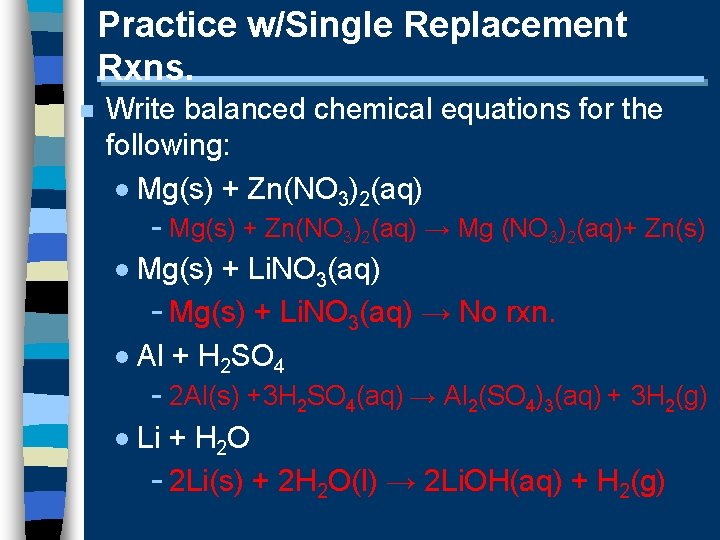Practice w/Single Replacement Rxns. n Write balanced chemical equations for the following: · Mg(s) + Zn(NO 3)2(aq) - Mg(s) + Zn(NO 3)2(aq) → Mg (NO 3)2(aq)+ Zn(s) · Mg(s) + Li. NO 3(aq) - Mg(s) + Li. NO 3(aq) → No rxn. · Al + H 2 SO 4 - 2 Al(s) +3 H 2 SO 4(aq) → Al 2(SO 4)3(aq) + 3 H 2(g) · Li + H 2 O - 2 Li(s) + 2 H 2 O(l) → 2 Li. OH(aq) + H 2(g)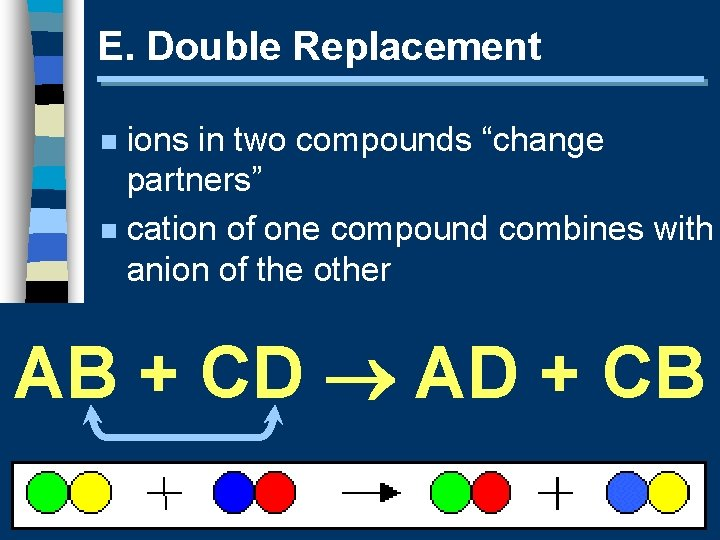E. Double Replacement ions in two compounds “change partners” n cation of one compound combines with anion of the other n AB + CD AD + CB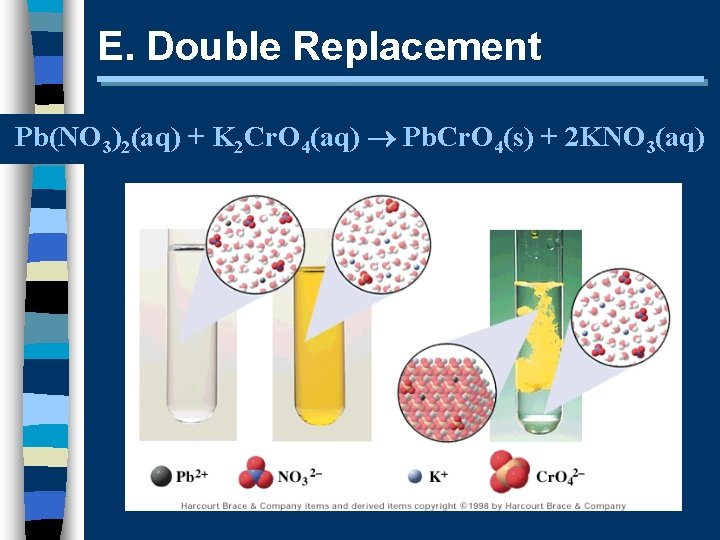E. Double Replacement Pb(NO 3)2(aq) + K 2 Cr. O 4(aq) Pb. Cr. O 4(s) + 2 KNO 3(aq)E. Double Replacement n Products: · switch negative ions · one product must be insoluble creates (s) or (l) (check solubility on your sheet) Pb(NO 3)2(aq)+2 KI(aq) Pb. I 2(s)+2 KNO 3(aq) Na. NO 3(aq)+ KI(aq) N. R.Practice with Double Displacement Rxns. n Write balanced chemical equations for the following: · HCl + Ca(OH)2 - 2 HCl(aq) + Ca(OH)2(s) → N. R. · KOH + H 3 PO 4 - 3 KOH(aq) + H 3 PO 4(aq) → K 3 PO 4(aq)+ 3 H 2 O(l) · Al(OH)3+ H 2 SO 4 - 2 Al(OH)3+ 3 H 2 SO 4(aq)→ Al 2(SO 4)3(aq)+ 6 H 2 O(l) · Sr. Br 2 + (NH 4)2 CO 3 - Sr. Br 2 + (NH 4)2 CO 3 → 2 NH 4 Br (aq) +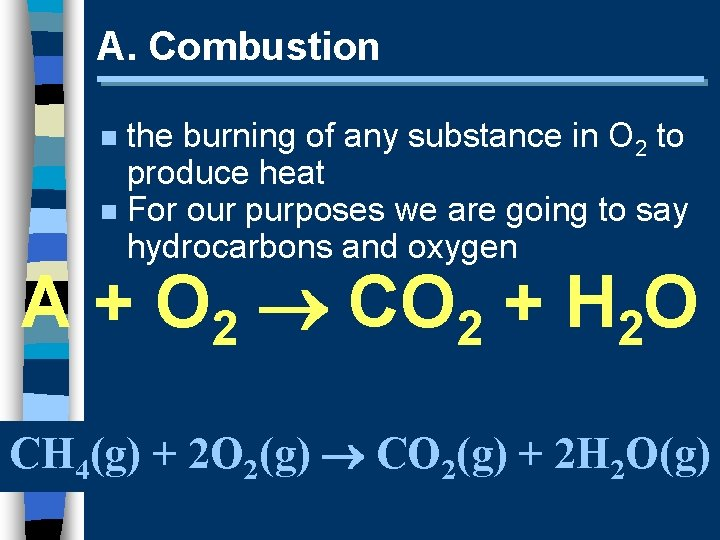A. Combustion the burning of any substance in O 2 to produce heat n For our purposes we are going to say hydrocarbons and oxygen n A + O 2 CO 2 + H 2 O CH 4(g) + 2 O 2(g) CO 2(g) + 2 H 2 O(g)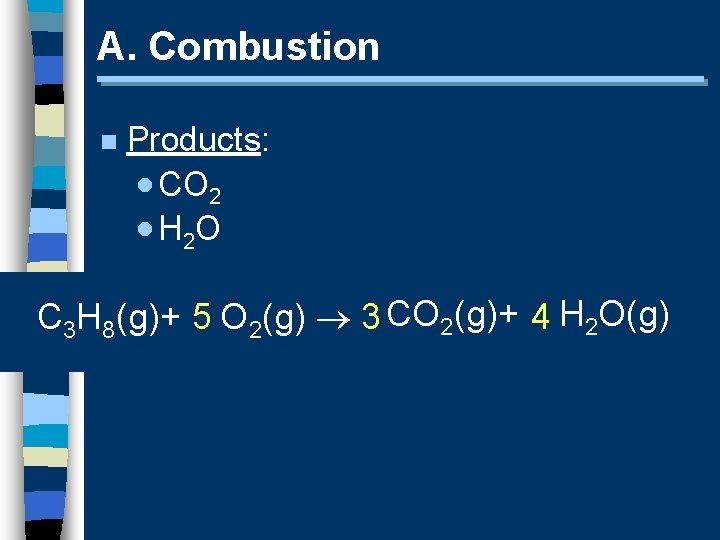A. Combustion n Products: · CO 2 · H 2 O C 3 H 8(g)+ 5 O 2(g) 3 CO 2(g)+ 4 H 2 O(g)Practice with combustion rxns. n Write a balanced equation for the complete combustion of the following: · Butene (g); C 4 H 8 - C 4 H 8(g) + 6 O 2(g) → 4 CO 2(g) + 4 H 2 O(g) · Glucose (s); C 6 H 12 O 6 - C 6 H 12 O 6(s) + 6 O 2(g)→ 6 CO 2(g)+ 6 H 2 O(g) · Octane (l); C 8 H 18 - 2 C 8 H 18(l) + 25 O 2 (g)→ 16 CO 2(g)+ 18 H 2 O(g)